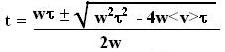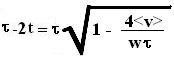Tuesday, July 10, 2007

Irodov Problem 1.3Mean velocity is total distance by total time. The v-t graph is shown in the figure beside.

Suppose that time of constant acceleration was t. During the initial acceleration phase thus the car will traveland its final speed will be. This can also be calculated using the v-t diagram as the area under the line AB (triangle ABE).

The car will take the same time t to come to complete rest and during this deceleration phase it will travel. This can be calculated in the v-t diagram as the area of the triangle CFD.

During the uniform motion phase the car travels at a speedand travels for the remaining time of. Thus , during the uniform motion phase it travels a distance of. This can be calculated as the area of the rectangle EBCF.

The total distance traveled is thus given by,The average velocity during the entire time is thus given by,The above equation is a quadratic equation which has two possible solutions,Clearly we choose the -ive sign since t cannot exceed the total time. The uniform interval is thus given by,Anonymous said...

we can do this problem more easily by drawing a v-t graphAnonymous said...

Thanks Irodov SirAnonymous said...

thanx for the solutions.
you are doin a great job.Anonymous said...

Muchas gracias desde España. Thank's from Spain.